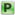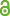Hauptmenü
• Autor
• Bazarova, Alina
• Berkes, István
• Horváth, Lajos
• TitelOn the Extremal Theory of Continued Fractions
• Datei
• DOI10.1007/s10959-014-0577-5
• Erschienen inJournal of Theoretical Probability
• Band29
• Erscheinungsjahr2016
• Heft1
• Seiten248-266
• LicenceCC BY
• ISSN1572-9230
• Zugriffsrechte• AbstractLetting $$x = [a 1 (x), a 2 (x), . . .]$$ denote the continued fraction expansion of an irrational 1 number $$x ∈ (0, 1)$$, Khinchin proved that $$S_n (x) = Σ^n_{k=1} a_k (x) ∼ \frac{1}{log 2} n log n$$ in measure, but not for almost every x. Diamond and Vaaler showed that removing the largest term from $$S_n (x)$$, the pre- vious asymptotics will hold almost everywhere, showing the crucial influence of the extreme terms of $$S_n (x)$$ on the sum. In this paper we determine, for $$d_n → ∞$$ and $$d_n/n → 0$$, the precise asymptotics of the sum of the $$d_n$$ largest terms of $$S_n (x)$$ and show that the sum of the remaining terms has an asymptotically Gaussian distribution.